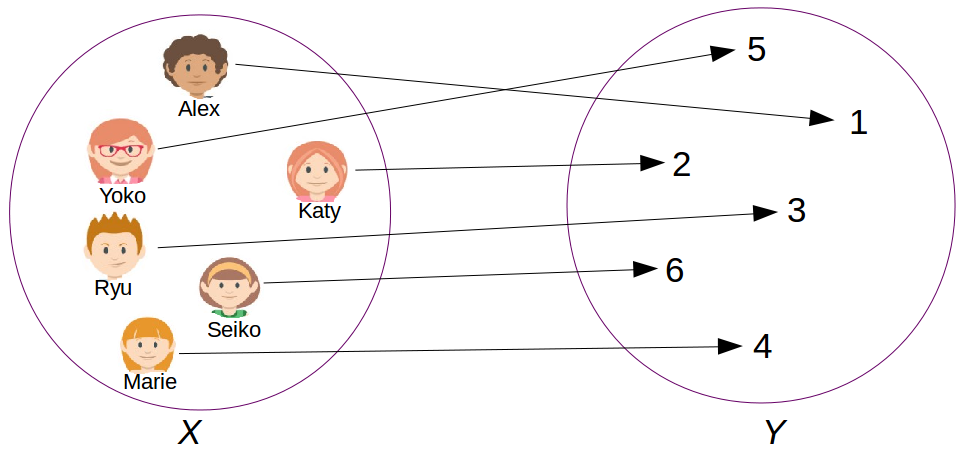## 1. Analogy

In basic algebra, the solution of the following equation is said to be the multiplicative inverse :

Solution of $(1)$ is $\frac{1}{a}$, which is also written $a^{-1}$.

In linear algebra, the equivalent equation to $(1)$ is :

In $(2)$, $I$ is the so-called identity matrix. It contains $1$’s in its diagonal and $0$’s everywhere else, as follows : $\left( \begin{smallmatrix} 1 & \cdots & 0 \\ \vdots & \phantom{/} 1 & \vdots \\ 0 & \cdots & 1 \end{smallmatrix} \right)$.

## 2. Calculation

The inverse matrix of $A=\left( \begin{smallmatrix} a_{1,1} & \cdots & a_{1,n} \\ \vdots & \cdots & \phantom{1}\vdots \\ a_{n,1} & \cdots & a_{n,n} \end{smallmatrix} \right)$ is obtained by dividing the transpose of the cofactor matrix, noted $C^{T}$, by the determinant of $A$ :

In $(3)$ there are two new concepts. First, the matrix of cofactors (or comatrix), second the transpose of a matrix.

Let’s first discuss on how to transpose a matrix. To transpose a matrix, convert its lines into columns.

#### Example I

Let $A=\left( \begin{smallmatrix} -5 & 0 \\ -8 & -1 \end{smallmatrix} \right)$. Let’s compute its transpose.

Let’s now tell more about the cofactor matrix. The cofactor $c_{i,j}$ of $a_{i,j}$ is defined as follows :

In $(5)$, $D_{i,j}$ is the determinant of $A$ without the row $\textbf{i}$ and without the column $\textbf{j}$.

#### Example II

Let $A=\left( \begin{smallmatrix} -5 & 0 \\ -8 & -1 \end{smallmatrix} \right)$. Let’s compute $D_{1,2}$ of $A$.

#### Example III

Let $A=\left( \begin{smallmatrix} 2 & 1 \\ 5 & -1 \end{smallmatrix} \right)$. Let’s compute its inverse.

First, let’s compute its determinant :

Second, let’s compute the cofactor matrix :

Third, let’s compute the transpose of $C$ and thus get the inverse of $A$:

## 3. Inverse function

More generally, the matrix inverse can be seen as an inverse function.

In analysis, an inverse function, noted $f^{-1}$, associates back the images with the elements in the starting set, as Figure 10.1 illustrates.

It turns out that a function has an inverse only if it is bijective.Figure 10.1 : inverse function (wikipedia)Figure 10.2 : bijection

A bijection is a function having a special property.
Any element from the starting set is associated, under the function, to one single element in the target set and vice versa. Figure 10.2 shows this property.

#### Example IV

Let $t$ :

$t$ is not bijective since many $\vec{x}$ are associated to $\vec{y}=\left( \begin{smallmatrix} 0 \\ 0 \end{smallmatrix} \right)$.
Indeed, by decomposing, $x_1 \left( \begin{smallmatrix} 2 \\ -6 \end{smallmatrix} \right) + x_2 \left( \begin{smallmatrix} 1 \\-3 \end{smallmatrix} \right) = \left( \begin{smallmatrix} 0 \\ 0 \end{smallmatrix} \right)$ has infinitely many solutions because the vectors are linearly dependent.

## Recapitulation

The matrix inverse of $A$, noted $A^{-1}$, has the following property : $AA^{-1} = A^{-1}A = I$.

A function $f$ has an inverse, noted $f^{-1}$, only if it is bijective. Therefore, for having an inverse a matrix $A$ have to satisfy the two following conditions :

• $A$ must be square
• columns of $A$ must be linearly independent

$A^{-1}$ is obtained by dividing the transpose of the cofactor matrix by the determinant of $A$, namely :